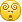# 1.变量

R：直接给变量赋值

```test = 1
```

Scala：val代表不可变，var代表可变，可以不写变量类型，scala会根据所赋的值进行推断

```val test:Int = 1
val test1 = 1
var test2 = 1
```

# 2.判断与循环

R：

```if (test > 0) {
print(test)
}

for (i in 1:10) {
print(i)
}
```

Scala：判断语句一样，循环语句也非常类似，像R一样，Scala可以通过to或者until函数非常方便地生成连续整数用于循环

```if (test > 0) {
print(test)
}

for (i <- 1 to 10) {
print(i)
}
```

# 3.函数

R：

```doubleX = function(x) {
x * 2
}
```

Scala：R与Scala都会将函数体中最后一个值作为返回值因此不需要写return；Scala中参数的类型是必须定义的而返回值的类型则不一定需要写（例外是递归函数，无法推断出返回值的类型）；与其它主流语言不同的是两者在函数定义中都有“=”，Scala的函数如果没有返回值（返回Unit）则可以省去“=”

```def doubleX(x:Double):Double = {
x * 2
}
```

# 4.集合

R：R语言里常用的集合就四种：vector, matrix, dataframe, list，写起来基本以开发效率优先，很少考虑数据结构与运行效率；从一个集合变换为另一个集合是常用操作，一般很少使用循环语句，取而代之的是sapply或者lapply

```# vector
c(1, 2, 3)

# matrix
matrix(1:10, nrow=2)

# dataframe
data.frame(x=1:3, y=4:6)

# list
list(1:4, c('a', 'b', 'c'))

# sapply
sapply(1:5, function(i) i*2)
```

Scala：运行在jvm里的Scala与Java语言兼容性非常好，拥有丰富的集合以及较为全面的数据结构，同时又不需要像Java那样写冗长的代码，可以说在开发效率与执行效率之间找到了一个不错的平衡点:## 4.1 Seq

Vector：以树形结构实现，支持快速的随机访问。

Range：前边提到的1 to 10生成的就是Range，并不存储所有值而只是起始值、结束值和增值。

List：根据When should I choose Vector in Scala?所说，List的优势在于head与tail方法，快速获取首个元素与其余子list，因此大多数情况下会选用Vector。

```# 创建对象
val test = Vector(1, 2, 3)

# 按下标选取值，从0开始
test(0)

# 集合变换
test.map(x => x * 2)
# 如果map中匿名函数的参数在=&gt;右侧只出现一次可以用_替换
test.map(_ * 2)
```

## 4.2 Set

HashSet：Set是不重复元素的集合，默认的Set是HashSet不保留插入的顺序，它的优势是查找元素的速度比Array和List快得多。

ListSet：维护一个链表使得元素插入的顺序可以被记住。

SortedSet：得到已排序的Set。

## 4.3 Map

Map是对偶（求英文名）或者键值对的集合，对偶是最简单的元组（tuple），元组是可以包含不同类型对象的集合。

```# 创建Map
val mapTest = Map('a'->1, 'b'->2, 'c'->3)

# 元组按下标选取值，从1开始
val test = 'a'->1
test._1

# 选出值<3的Map子集
mapTest.filter(_._2 < 3)
```

# 5.面向对象

R：R常被用来写脚本，较少用于庞大的项目，因此面相对象的用法较少，在工作中应用过S4的方法，但说实在的语法真的挺奇怪的，用起来不舒服。

Scala：作为Java的兄弟，Scala的面向对象编程可谓非常完整，这里记录一些Scala区别于Java的地方

## 5.1 class

```class Person(var age: Int) {
}

var person = new Person(10)
person.age = 20
println(person.age)
```
• 与Java不同，Scala可以像给函数定义参数一样给类定义字段，这被称为主构造器
• Scala会自动为类生成setter和getter，上面例子中对age的取值与赋值其实是通过setter与getter方法实现的

## 5.2 object

• Scala不能在类中定义静态方法与静态字段，不过可以通过object来近似实现
• object不能提供构造器
• 与class同名且在同一个源文件中的object，可以与类互相访问私有特性，被称作伴生对象
• 初始化集合对象时，如：Vector(1,2,3)，其实是调用了Vector object的apply方法
• 每个Scala程序都必须从一个object的main方法开始，这个object就是应用程序对象
• 用obect可以实现枚举
• unapply是一个接收对象，返回构造参数的方法，定义了这个方法的object被称作提取器

## 5.3 trait

• 可以把它当成能够有方法实现的接口

# 6.模式匹配

## 6.1 switch中的模式

```# if守卫
ch match {
case '+' => sigh = 1
case '-' => sigh = -1
case _ if Character.isDigit(ch) => digit = Character.digit(ch, 10)
case _ => sigh = 0
}

# 类型匹配（不需要用asInstanceOf）
obj match {
case x:Int => x
case s:String => Interger.parseInt(s)
case _:BigInt => Int.MaxValue
case _ => 0
}

# 匹配数组、列表和元组
arr match {
case Array(0) => "0"
case Array(x, y) => x + " " + y
case Array(0, _*) => "0 ..."
case _ => "something else"
}
```

## 6.2 变量声明中的模式

```scala> val (x, y) = (1, 2)
x: Int = 1
y: Int = 2

scala> val (q, r) = BigInt(10) /% 3
q: scala.math.BigInt = 3
r: scala.math.BigInt = 1

scala> var Array(first, seccond, _*) = Array(1,2,3)
first: Int = 1
seccond: Int = 2

# 正则表达式
scala> val numitemPattern = "(\\d+) (\\w+)".r
numitemPattern: scala.util.matching.Regex = (\d+) (\w+)

scala> val numitemPattern(num, item) = "99 bottles"
num: String = 99
item: String = bottles
```

## 6.3 for表达式中的模式

```# 打印所有值为空白的键
for ((k, "") <- System.getProperties()) println(k)
```

## 《R语言码农的Scala学习心得》上有5条评论

1.肖俊说道：

写起来是不是很High~早叫你学scala了，非要用R折腾SPARK啊。总算迷途知返啊1.Logos说道：

哈哈哈，还是原生语言靠谱

2.徐飏说道：

我也是一直在spark上用R，其实用sparklyr包可以满足大部分spark上的操作的，就是各种莫名其妙的问题层出不穷。现在也开始转向scala，感觉抛开对数据类型的操作不提的话，就spark本身的api而言，比R方便一些，但也没有轻松太多……看来还是学艺不精1.Logos说道：

是会有各种莫名其妙的问题，习惯一下Scala一切就都顺利了，我现在只用SparkR做一些数据清洗和整理的工作，同时导出Spark能读取的parquet格式。具体的模型训练和调参还是用Scala比较稳

3.徐飏说道：